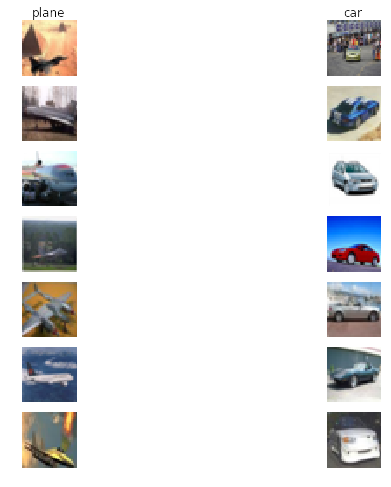## Logistic regression

### Building a logistic regression classifier to distinguish 'plane' and 'car' in CIFAR-10 dataset

• implement a fully-vectorized loss function for the Logistic Regression
• implement the fully-vectorized expression for its analytic gradient
• check implementation using numerical gradient
• use a validation set to tune the learning rate and regularization strength
• optimize the loss function with Batch Gradient Descent and Stochastic Gradient Descent
In :
# Setup code for this notebook
import random
import numpy as np
import matplotlib.pyplot as plt

# This is a bit of magic gto make matplotlib figures appear inline
# in the notebook rather than in a new window
%matplotlib inline
plt.rcParams['figure.figsize'] = (10.0, 8.0)
plt.rcParams['image.interpolation'] = 'nearest'
plt.rcParams['image.cmap'] = 'gray'
# Some more magic so that the notebook will reload external python modules;


get_datasets.sh

# Get CIFAR10
wget http://www.cs.toronto.edu/~kriz/cifar-10-python.tar.gz
tar -xzvf cifar-10-python.tar.gz
rm cifar-10-python.tar.gz### Write function to load data in data_utils.py

In :
# Write function to load the cifar-10 data
# The original code is from http://cs231n.github.io/assignment1/
# The function is in data_utils.py file for reusing.
import cPickle as pickle
import numpy as np
import os

""" load single batch of cifar """
with open(filename, 'r') as f:
X = X.reshape(10000, 3, 32, 32).transpose(0,2,3,1).astype("float")
Y = np.array(Y)
return X, Y

""" load all of cifar """
xs = []
ys = []
for b in range(1,6):
f = os.path.join(ROOT, 'data_batch_%d' % (b, ))
xs.append(X)
ys.append(Y)
Xtr = np.concatenate(xs)
Ytr = np.concatenate(ys)
del X, Y
return Xtr, Ytr, Xte, Yte


## Load data and visualize some samples

In :
from algorithms.data_utils import load_CIFAR10
classes = ['plane', 'car', 'bird', 'cat', 'deer', 'dog', 'frog', 'horse', 'ship', 'truck']

def get_CIFAR10_data(num_training=49000, num_val=1000, num_test=10000, show_sample=True):
"""
Load the CIFAR-10 dataset, and divide the sample into training set, validation set and test set
"""

cifar10_dir = 'datasets/datasets-cifar-10/cifar-10-batches-py/'
X_train, y_train, X_test, y_test = load_CIFAR10(cifar10_dir)

# subsample the data for validation set
mask = xrange(num_training, num_training + num_val)
return X_train, y_train, X_val, y_val, X_test, y_test

def subset_classes_data(classes):
# Subset 'plane' and 'car' classes to perform logistic regression
idxs = np.logical_or(y_train_raw == 0, y_train_raw == 1)
X_train = X_train_raw[idxs, :]
y_train = y_train_raw[idxs]
# validation set
idxs = np.logical_or(y_val_raw == 0, y_val_raw == 1)
X_val = X_val_raw[idxs, :]
y_val = y_val_raw[idxs]
# test set
idxs = np.logical_or(y_test_raw == 0, y_test_raw == 1)
X_test = X_test_raw[idxs, :]
y_test = y_test_raw[idxs]
return X_train, y_train, X_val, y_val, X_test, y_test

def visualize_sample(X_train, y_train, classes, samples_per_class=7):
"""visualize some samples in the training datasets """
num_classes = len(classes)
for y, cls in enumerate(classes):
idxs = np.flatnonzero(y_train == y) # get all the indexes of cls
idxs = np.random.choice(idxs, samples_per_class, replace=False)
for i, idx in enumerate(idxs): # plot the image one by one
plt_idx = i * num_classes + y + 1 # i*num_classes and y+1 determine the row and column respectively
plt.subplot(samples_per_class, num_classes, plt_idx)
plt.imshow(X_train[idx].astype('uint8'))
plt.axis('off')
if i == 0:
plt.title(cls)
plt.show()

def preprocessing_CIFAR10_data(X_train, y_train, X_val, y_val, X_test, y_test):

# Preprocessing: reshape the image data into rows
X_train = np.reshape(X_train, (X_train.shape, -1)) # [49000, 3072]
X_val = np.reshape(X_val, (X_val.shape, -1)) # [1000, 3072]
X_test = np.reshape(X_test, (X_test.shape, -1)) # [10000, 3072]

# Normalize the data: subtract the mean image
mean_image = np.mean(X_train, axis = 0)
X_train -= mean_image
X_val -= mean_image
X_test -= mean_image

# Add bias dimension and transform into columns
X_train = np.hstack([X_train, np.ones((X_train.shape, 1))]).T
X_val = np.hstack([X_val, np.ones((X_val.shape, 1))]).T
X_test = np.hstack([X_test, np.ones((X_test.shape, 1))]).T
return X_train, y_train, X_val, y_val, X_test, y_test

# Invoke the above functions to get our data
X_train_raw, y_train_raw, X_val_raw, y_val_raw, X_test_raw, y_test_raw = get_CIFAR10_data()
visualize_sample(X_train_raw, y_train_raw, classes)
subset_classes = ['plane', 'car']
X_train_raw, y_train_raw, X_val_raw, y_val_raw, X_test_raw, y_test_raw = subset_classes_data(subset_classes)
X_train, y_train, X_val, y_val, X_test, y_test = preprocessing_CIFAR10_data(X_train_raw, y_train_raw, X_val_raw, y_val_raw, X_test_raw, y_test_raw)

# As a sanity check, we print out th size of the training and test data dimenstion
print 'Train data shape: ', X_train.shape
print 'Train labels shape: ', y_train.shape
print 'Validation data shape: ', X_val.shape
print 'Validation labels shape: ', y_val.shape
print 'Test data shape: ', X_test.shape
print 'Test labels shape: ', y_test.shape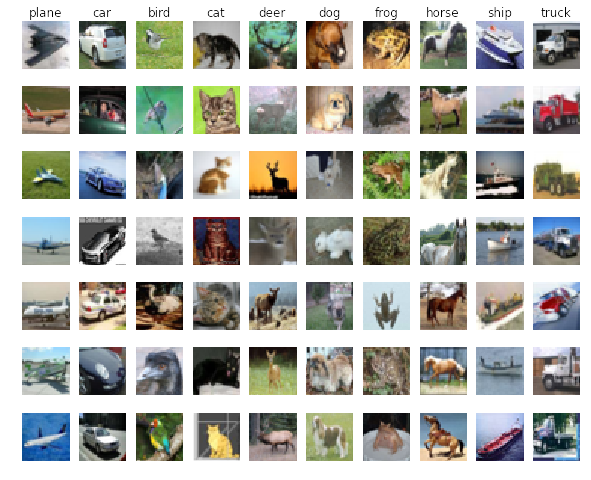Train data shape:  (3073, 9794)
Train labels shape:  (9794,)
Validation data shape:  (3073, 206)
Validation labels shape:  (206,)
Test data shape:  (3073, 2000)
Test labels shape:  (2000,)



## Logisic Regression Classifier

The code is running in the backend, you can find it here, or github

In :
# Test the loss and gradient and compare between two implementations
import time

# generate a rand weights W
W = np.random.randn(1, X_train.shape) * 0.001
tic = time.time()
toc = time.time()
print 'Naive loss: %f, and gradient: computed in %fs' % (loss_naive, toc - tic)

tic = time.time()
toc = time.time()
print 'Vectorized loss: %f, and gradient: computed in %fs' % (loss_vec, toc - tic)

# Compare the gradient, because the gradient is a vector, we canuse the Frobenius norm to compare them
# the Frobenius norm of two matrices is the square root of the squared sum of differences of all elements
# Randomly choose some gradient to check
idxs = np.random.choice(X_train.shape, 10, replace=False)
print idxs
print 'Gradient difference between naive and vectorized version is: %f' % diff

Naive loss: -0.012129, and gradient: computed in 12.395058s
Vectorized loss: 1.733340, and gradient: computed in 0.030889s
[2215 1691 2497 1324   85 2936 1186 1656  633 1815]
[  1.20943239  -2.95093674  -4.75710499  -2.24515916 -11.93801341
2.34233898  -3.89090938  -4.50029132  -9.25694246  -0.88358345]
[  1.20943239  -2.95093674  -4.75710499  -2.24515916 -11.93801341
2.34233898  -3.89090938  -4.50029132  -9.25694246  -0.88358345]
Gradient difference between naive and vectorized version is: 0.000000



## Write function to compute gradient numerically for test the analytic gradient

And put the function in algorithms/gradient_check.py file for future use

In :
# file: algorithms/gradient_check.py
"""
sample a few random elements and only return numerical
in this dimensions.
"""
h = 1e-5

print x.shape

for i in xrange(num_checks):
ix = tuple([randrange(m) for m in x.shape])
print ix
x[ix] += h # increment by h
fxph = f(x) # evaluate f(x + h)
x[ix] -= 2 * h # increment by h
fxmh = f(x) # evaluate f(x - h)
x[ix] += h # reset

grad_numerical = (fxph - fxmh) / (2 * h)
print 'numerical: %f analytic: %f, relative error: %e' % (grad_numerical, grad_analytic, rel_error)

In :
# Check gradient using numerical gradient along several randomly chosen dimenstion
f = lambda w: loss_grad_logistic_vectorized(w, X_train, y_train, 0)

(1, 3073)
(0, 3063)
numerical: -4.950364 analytic: -4.950364, relative error: 2.766216e-09
(0, 1816)
numerical: 1.432762 analytic: 1.432762, relative error: 1.279659e-08
(0, 1958)
numerical: 0.047512 analytic: 0.047512, relative error: 3.384999e-07
(0, 2453)
numerical: 7.979511 analytic: 7.979511, relative error: 2.289589e-09
(0, 1241)
numerical: -2.151217 analytic: -2.151218, relative error: 1.386304e-08
(0, 1554)
numerical: -4.431215 analytic: -4.431215, relative error: 4.006368e-10
(0, 747)
numerical: -8.951594 analytic: -8.951594, relative error: 3.506487e-09
(0, 414)
numerical: -16.110520 analytic: -16.110520, relative error: 1.739342e-09
(0, 2892)
numerical: -3.647348 analytic: -3.647348, relative error: 1.891536e-09
(0, 2287)
numerical: 7.350139 analytic: 7.350139, relative error: 2.401293e-09


In :
# Training logistic regression classifier using SGD
from algorithms.classifiers import Logistic

# using BGD algorithm
logistic_bgd = Logistic()
tic = time.time()
losses_bgd = logistic_bgd.train(X_train, y_train, method='bgd', batch_size=200, learning_rate=1e-6,
reg = 1e2, num_iters=1000, verbose=True, vectorized=True)
toc = time.time()
print 'Traning time for BGD with vectorized version is %f \n' % (toc - tic)

# Compute the accuracy of training data and validation data using Logistic.predict function
y_train_pred_bgd = logistic_bgd.predict(X_train)
print 'Training accuracy: %f' % (np.mean(y_train == y_train_pred_bgd))
y_val_pred_bgd = logistic_bgd.predict(X_val)
print 'Validation accuracy: %f' % (np.mean(y_val == y_val_pred_bgd))

# # using SGD algorithm
logistic_sgd = Logistic()
tic = time.time()
losses_sgd = logistic_sgd.train(X_train, y_train, method='sgd', batch_size=200, learning_rate=1e-6,
reg = 1e2, num_iters=1000, verbose=True, vectorized=True)
toc = time.time()
print 'Traning time for SGD with vectorized version is %f \n' % (toc - tic)

y_train_pred_sgd = logistic_sgd.predict(X_train)
print 'Training accuracy: %f' % (np.mean(y_train == y_train_pred_sgd))
y_val_pred_sgd = logistic_sgd.predict(X_val)
print 'Validation accuracy: %f' % (np.mean(y_val == y_val_pred_sgd))

iteration 0/1000: loss 1.483346
iteration 100/1000: loss 0.701950
iteration 200/1000: loss 0.641996
iteration 300/1000: loss 0.610521
iteration 400/1000: loss 0.589423
iteration 500/1000: loss 0.573713
iteration 600/1000: loss 0.561257
iteration 700/1000: loss 0.550941
iteration 800/1000: loss 0.542122
iteration 900/1000: loss 0.534400
Traning time for BGD with vectorized version is 32.749114

Training accuracy: 0.817643
Validation accuracy: 0.796117
iteration 0/1000: loss 1.571740
iteration 100/1000: loss 0.663951
iteration 200/1000: loss 0.639361
iteration 300/1000: loss 0.617110
iteration 400/1000: loss 0.553394
iteration 500/1000: loss 0.612563
iteration 600/1000: loss 0.509184
iteration 700/1000: loss 0.522065
iteration 800/1000: loss 0.643715
iteration 900/1000: loss 0.490812
Traning time for SGD with vectorized version is 4.026003

Training accuracy: 0.810905
Validation accuracy: 0.810680


In :
# A useful degugging strategy is to plot the loss
# as a function of iteration number:
from ggplot import *
qplot(xrange(len(losses_bgd)), losses_bgd) + labs(x='Iteration number', y='BGD Loss value')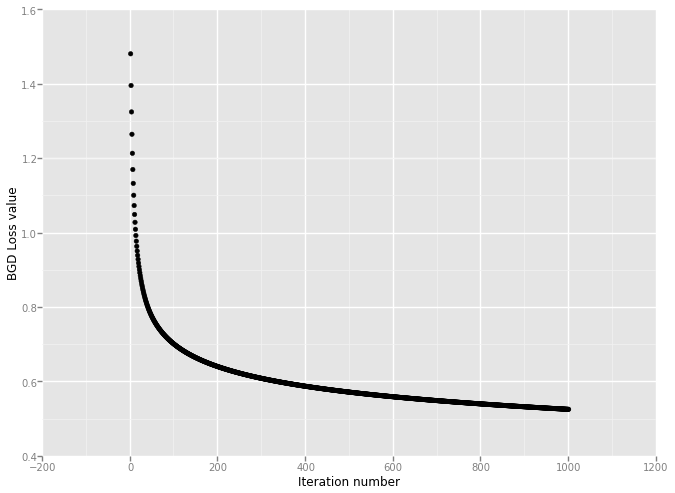Out:
<ggplot: (288057697)>

In :
qplot(xrange(len(losses_sgd)), losses_sgd) + labs(x='Iteration number', y='SGD Loss value')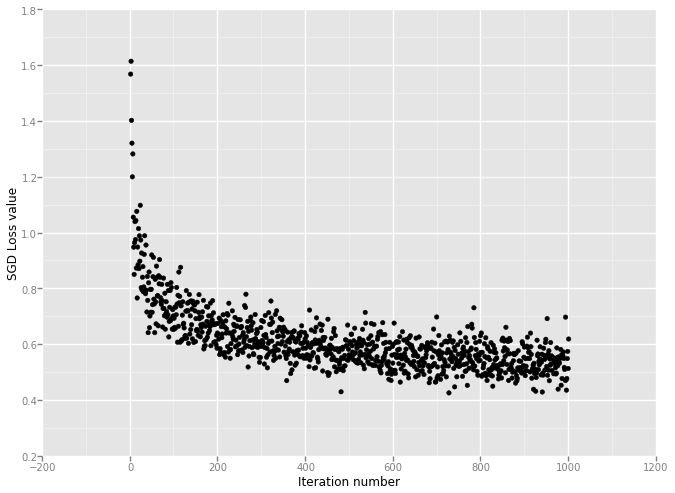Out:
<ggplot: (286135049)>


## A little summary

From the computing results, we can see that: * Both the training accuracy and validation accuracy for SGD and BGD can reach 80%. * The loss decreasing of SGD is much faster than BGD. * Based on the selected learning rate and regularization strength, BGD needs more time to converge. * There is not easy way to determine the learning rate and regularization strength. Firstly I use large learning rate, and the loss goes up and there are some 'nan' because some numbers are too big.

In :
# Using validation set to tuen hyperparameters, i.e., learning rate and regularization strength
learning_rates = [1e-5, 1e-8]
regularization_strengths = [10e2, 10e4]

# Result is a dictionary mapping tuples of the form (learning_rate, regularization_strength)
# to tuples of the form (training_accuracy, validation_accuracy). The accuracy is simply the fraction
# of data points that are correctly classified.
results = {}
best_val = -1
best_logistic = None

# Choose the best hyperparameters by tuning on the validation set
i = 0
interval = 5
for learning_rate in np.linspace(learning_rates, learning_rates, num=interval):
i += 1
print 'The current iteration is %d/%d' % (i, interval)
for reg in np.linspace(regularization_strengths, regularization_strengths, num=interval):
logistic = Logistic()
logistic.train(X_train, y_train, method='sgd', batch_size=200, learning_rate=learning_rate,
reg = reg, num_iters=1000, verbose=False, vectorized=True)
y_train_pred = logistic.predict(X_train)
y_val_pred = logistic.predict(X_val)
train_accuracy = np.mean(y_train == y_train_pred)
val_accuracy = np.mean(y_val == y_val_pred)
results[(learning_rate, reg)] = (train_accuracy, val_accuracy)
if val_accuracy > best_val:
best_val = val_accuracy
best_logistic = logistic
else:
pass

# Print out the results
for learning_rate, reg in sorted(results):
train_accuracy,val_accuracy = results[(learning_rate, reg)]
print 'learning rate %e and regularization %e, \n \
the training accuracy is: %f and validation accuracy is: %f.\n' % (learning_rate, reg, train_accuracy, val_accuracy)


The current iteration is 1/5
The current iteration is 2/5
The current iteration is 3/5
The current iteration is 4/5
The current iteration is 5/5
learning rate 1.000000e-08 and regularization 1.000000e+03,
the training accuracy is: 0.668164 and validation accuracy is: 0.621359.

learning rate 1.000000e-08 and regularization 2.575000e+04,
the training accuracy is: 0.645701 and validation accuracy is: 0.626214.

learning rate 1.000000e-08 and regularization 5.050000e+04,
the training accuracy is: 0.672861 and validation accuracy is: 0.650485.

learning rate 1.000000e-08 and regularization 7.525000e+04,
the training accuracy is: 0.684603 and validation accuracy is: 0.689320.

learning rate 1.000000e-08 and regularization 1.000000e+05,
the training accuracy is: 0.680417 and validation accuracy is: 0.718447.

learning rate 2.507500e-06 and regularization 1.000000e+03,
the training accuracy is: 0.817643 and validation accuracy is: 0.805825.

learning rate 2.507500e-06 and regularization 2.575000e+04,
the training accuracy is: 0.788340 and validation accuracy is: 0.800971.

learning rate 2.507500e-06 and regularization 5.050000e+04,
the training accuracy is: 0.770268 and validation accuracy is: 0.776699.

learning rate 2.507500e-06 and regularization 7.525000e+04,
the training accuracy is: 0.782009 and validation accuracy is: 0.781553.

learning rate 2.507500e-06 and regularization 1.000000e+05,
the training accuracy is: 0.646825 and validation accuracy is: 0.640777.

learning rate 5.005000e-06 and regularization 1.000000e+03,
the training accuracy is: 0.822544 and validation accuracy is: 0.815534.

learning rate 5.005000e-06 and regularization 2.575000e+04,
the training accuracy is: 0.617113 and validation accuracy is: 0.601942.

learning rate 5.005000e-06 and regularization 5.050000e+04,
the training accuracy is: 0.706861 and validation accuracy is: 0.723301.

learning rate 5.005000e-06 and regularization 7.525000e+04,
the training accuracy is: 0.694915 and validation accuracy is: 0.708738.

learning rate 5.005000e-06 and regularization 1.000000e+05,
the training accuracy is: 0.694405 and validation accuracy is: 0.708738.

learning rate 7.502500e-06 and regularization 1.000000e+03,
the training accuracy is: 0.799061 and validation accuracy is: 0.781553.

learning rate 7.502500e-06 and regularization 2.575000e+04,
the training accuracy is: 0.778129 and validation accuracy is: 0.786408.

learning rate 7.502500e-06 and regularization 5.050000e+04,
the training accuracy is: 0.775679 and validation accuracy is: 0.786408.

learning rate 7.502500e-06 and regularization 7.525000e+04,
the training accuracy is: 0.688585 and validation accuracy is: 0.713592.

learning rate 7.502500e-06 and regularization 1.000000e+05,
the training accuracy is: 0.372575 and validation accuracy is: 0.364078.

learning rate 1.000000e-05 and regularization 1.000000e+03,
the training accuracy is: 0.811517 and validation accuracy is: 0.805825.

learning rate 1.000000e-05 and regularization 2.575000e+04,
the training accuracy is: 0.699612 and validation accuracy is: 0.733010.

learning rate 1.000000e-05 and regularization 5.050000e+04,
the training accuracy is: 0.412191 and validation accuracy is: 0.412621.

learning rate 1.000000e-05 and regularization 7.525000e+04,
the training accuracy is: 0.386053 and validation accuracy is: 0.359223.

learning rate 1.000000e-05 and regularization 1.000000e+05,
the training accuracy is: 0.356034 and validation accuracy is: 0.354369.



## Compare the performance with and without regularization

From the results below, we can see that most the time, unregularzied version works better than unregularized one. However, some times it doesn't. In my opinion, it could be affected by the initialization.

In :
# Iterate the process num_iter times
num_iter = 5
reg_val_accu = []
unreg_val_accu = []
reg_test_accu = []
unreg_test_accu = []

for i in xrange(num_iter):
print  'The iteration %d/%d' % (i, num_iter)
logistic_reg = Logistic()
logistic_unreg = Logistic()
losses_temp1 = logistic_reg.train(X_train, y_train, method='sgd', batch_size=200, learning_rate=2.507500e-06,
reg = 1.000000e+03, num_iters=1000, verbose=False, vectorized=True)

losses_temp2 = logistic_unreg.train(X_train, y_train, method='sgd', batch_size=200, learning_rate=2.507500e-06,
reg = 0, num_iters=1000, verbose=False, vectorized=True)

y_val_pred_reg = logistic_reg.predict(X_val)
y_val_pred_unreg = logistic_unreg.predict(X_val)
y_test_pred_reg = logistic_reg.predict(X_test)
y_test_pred_unreg = logistic_unreg.predict(X_test)
reg_val_accu.append(np.mean(y_val_pred_reg == y_val))
unreg_val_accu.append(np.mean(y_val_pred_unreg == y_val))
reg_test_accu.append(np.mean(y_test_pred_reg == y_test))
unreg_test_accu.append(np.mean(y_test_pred_unreg == y_test))

print 'Regularized validation accuracy: ', reg_val_accu
print 'Unregularized validation accuracy: ', unreg_val_accu
print 'Regularized test accuracy: ', reg_test_accu
print 'Unregularized test accuracy: ', unreg_test_accu

The iteration 0/5
The iteration 1/5
The iteration 2/5
The iteration 3/5
The iteration 4/5
Regularized validation accuracy:  [0.82524271844660191, 0.81067961165048541, 0.80582524271844658, 0.82038834951456308, 0.80582524271844658]
Unregularized validation accuracy:  [0.82524271844660191, 0.82524271844660191, 0.82524271844660191, 0.83009708737864074, 0.77184466019417475]
Regularized test accuracy:  [0.82699999999999996, 0.82799999999999996, 0.83099999999999996, 0.82150000000000001, 0.82699999999999996]
Unregularized test accuracy:  [0.81399999999999995, 0.81200000000000006, 0.81499999999999995, 0.8175, 0.80049999999999999]



## Test best logistic classifier on test datasets

The trained model works well on the test datasets, which has 80% accuracy!

In :
y_test_predict_result = best_logistic.predict(X_test)
y_test_predict = y_test_predict_result
test_accuracy = np.mean(y_test == y_test_predict)
print 'The test accuracy is: %f' % test_accuracy
y_test_scores = y_test_predict_result
print y_test_scores
qplot(xrange(len(y_test_scores)), y_test_scores) + labs(x='Sample number', y='Scores')

The test accuracy is: 0.829500
[ 0.11488993  0.89215468  0.98832917 ...,  0.76654904  0.25399418
0.5842926 ]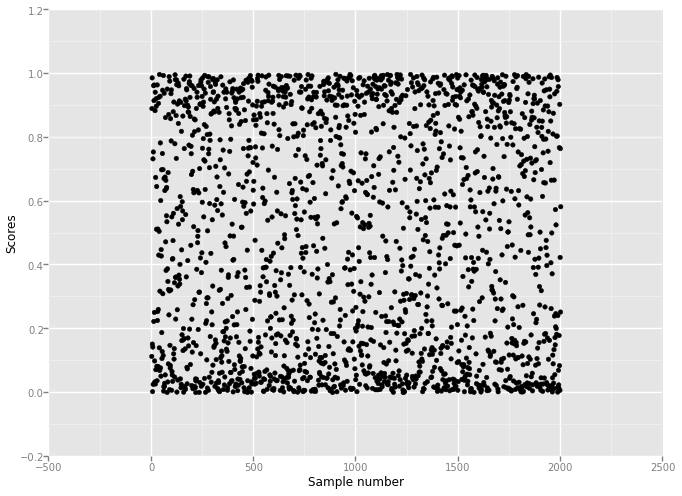Out:
<ggplot: (380076917)>


## Show the sample images which were not correctly classified

In :
idx_wrong = np.flatnonzero(y_test != y_test_predict) # 1-dimenstion array
X_test_wrong = X_test_raw[idx_wrong]
y_test_wrong = y_test_raw[idx_wrong]
X_test_right = X_test_raw[-idx_wrong]
y_test_right = y_test_raw[-idx_wrong]
scores_wrong = y_test_scores[idx_wrong]
scores_right = y_test_scores[-idx_wrong]
print X_test_wrong.shape
print X_test_raw.shape
print y_test_wrong.shape
print y_test_raw.shape
qplot(xrange(len(scores_wrong)), scores_wrong) + labs(x='Sample number', y='Wrong Scores')
qplot(xrange(len(scores_right)), scores_right) + labs(x='Sample number', y='Right Scores')

(341, 32, 32, 3)
(2000, 32, 32, 3)
(341,)
(2000,)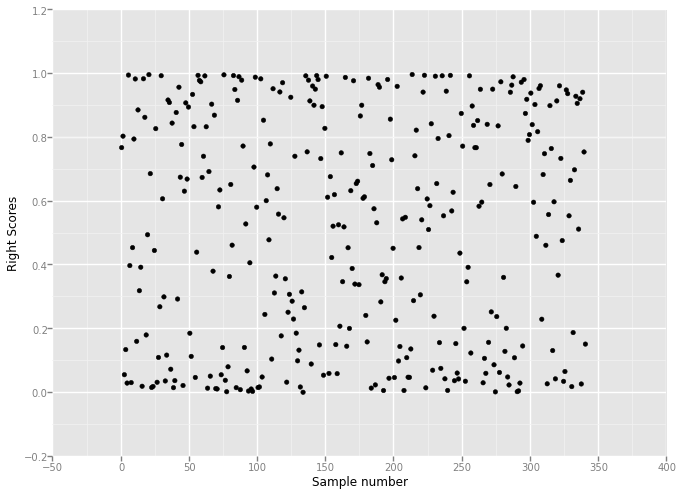Out:
<ggplot: (286843317)>

In :
# show the first 10 image
# clases = ['plane', 'car']
classes = ['plane', 'car']
visualize_sample(X_test_wrong, y_test_wrong, classes)
visualize_sample(X_test_right, y_test_right, classes)